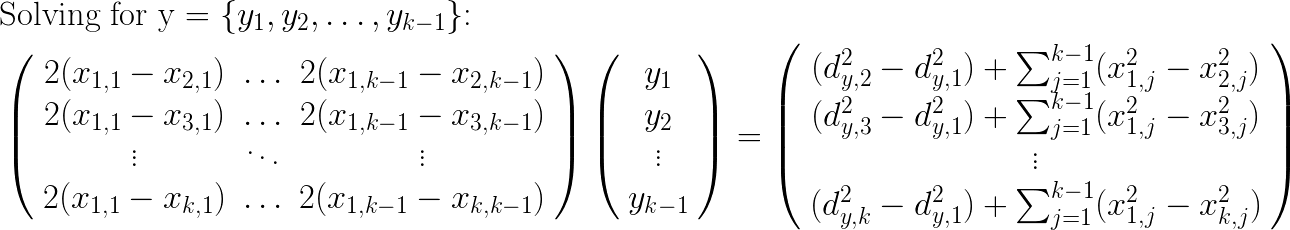Suppose I have a collection of (N) objects, and distances d(j,k) between each pair of objects (j) and (k); that is, my objects are members of a metric space. I have no knowledge about my objects, beyond these pair-wise distances. These objects could be construed as vertices in an (N-1) dimensional simplex. However, since I have no spatial information about my objects, I first need a way to assign spatial locations to each object, in vector space R^(N-1), with only my object distances to work with.

In this post I will derive an algorithm for assigning vertex locations in R^(N-1) for each of N objects, using only pairwise object distances.

I will assume that N >= 2, since at least two object are required to define a pairwise distance. The case N=2 is easy, as I can assign vertex 1 to the origin, and vertex 2 to the point d(1,2), to form a 1-simplex (i.e. a line segment) whose single edge is just the distance between the two objects. I will also assume that my N objects are distinct; that is, each pair has a non-zero distance.

Next consider an arbitrary N, and suppose I have already added vertices 1 through k. The next vertex (k+1) must obey the pairwise distance relations, as follows:Adding the new vertex (k+1) involves adding another dimension (k) to the simplex. I define this new kth coordinate x(k) to be zero for the existing k vertices, as annotated above; only the new vertex (k+1) will have a non-zero kth coordinate. Expanding the quadratic terms on the left yields the following form: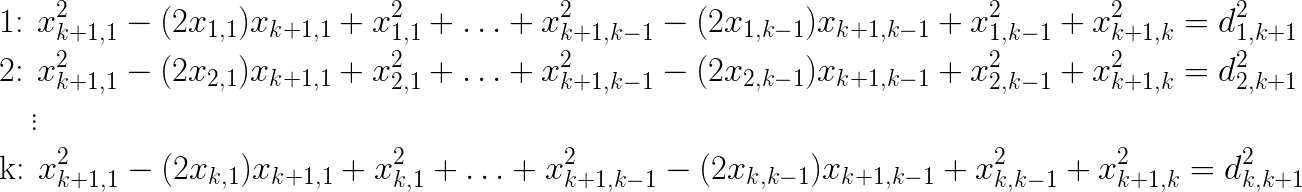The squared terms for the coordinates of the new vertex (k+1) are inconvenient, however I can get rid of them by subtracting pairs of equations above. For example, if I subtract equation 1 from the remaining k-1 equations (2 through k), these squared terms disappear, leaving me with the following system of k-1 equations, which we can see is linear in the 1st k-1 coordinates of the new vertex. Therefore, I know I’ll be able to solve for those coordinates. I can solve for the remaining kth coordinate by plugging it into the first distance equation: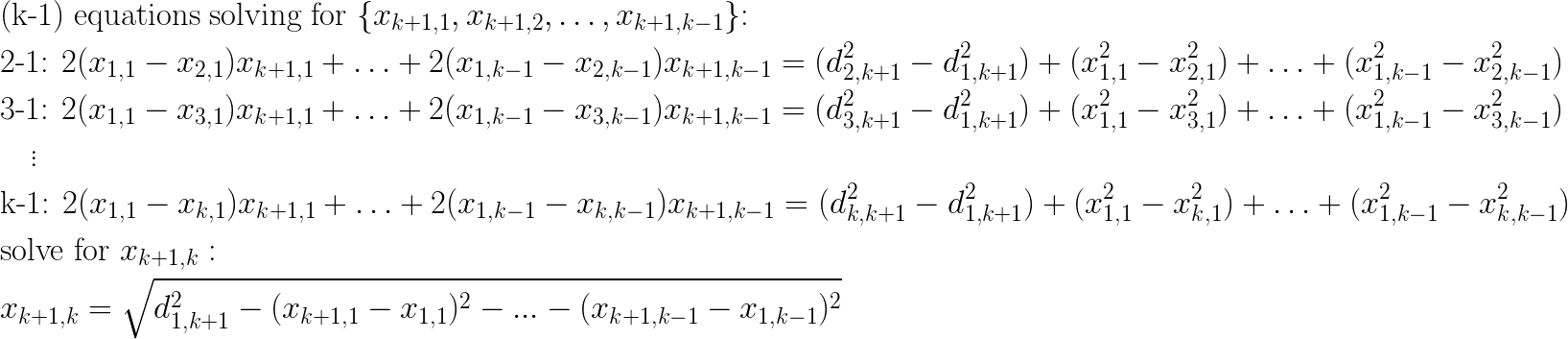To clarify matters, the equations above can be re-written as the following matrix equation, solveable by any linear systems library: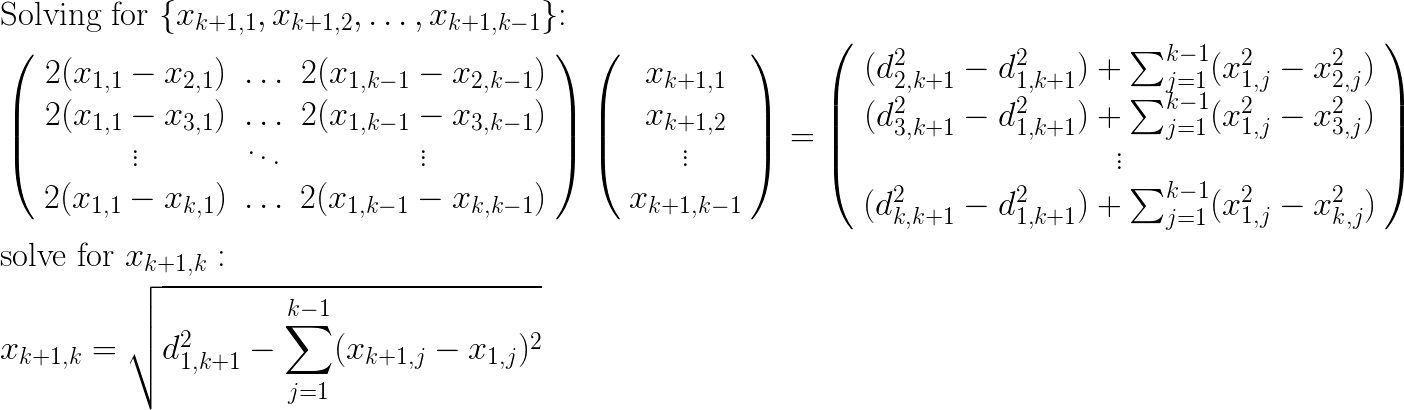This gives me a recusion relation for adding a new vertex (k+1), given that I have already added the first k vertices. The basis case of adding the first two vertices was already described above. And so I can iteratively add all my vertices one at a time by applying the recursion relation.

As a corollary, assume that I have constructed a simplex having k vertices, as shown above, and I would like to assign a spatial location to a new object, (y), given its k distances to each vertex. The corresponding distance relations are given by: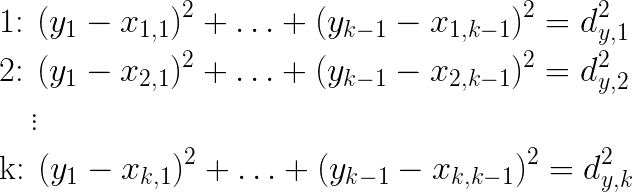I can apply a derivation very similar to the one above, to obtain the following linear equation for the (k-1) coordinates of (y):